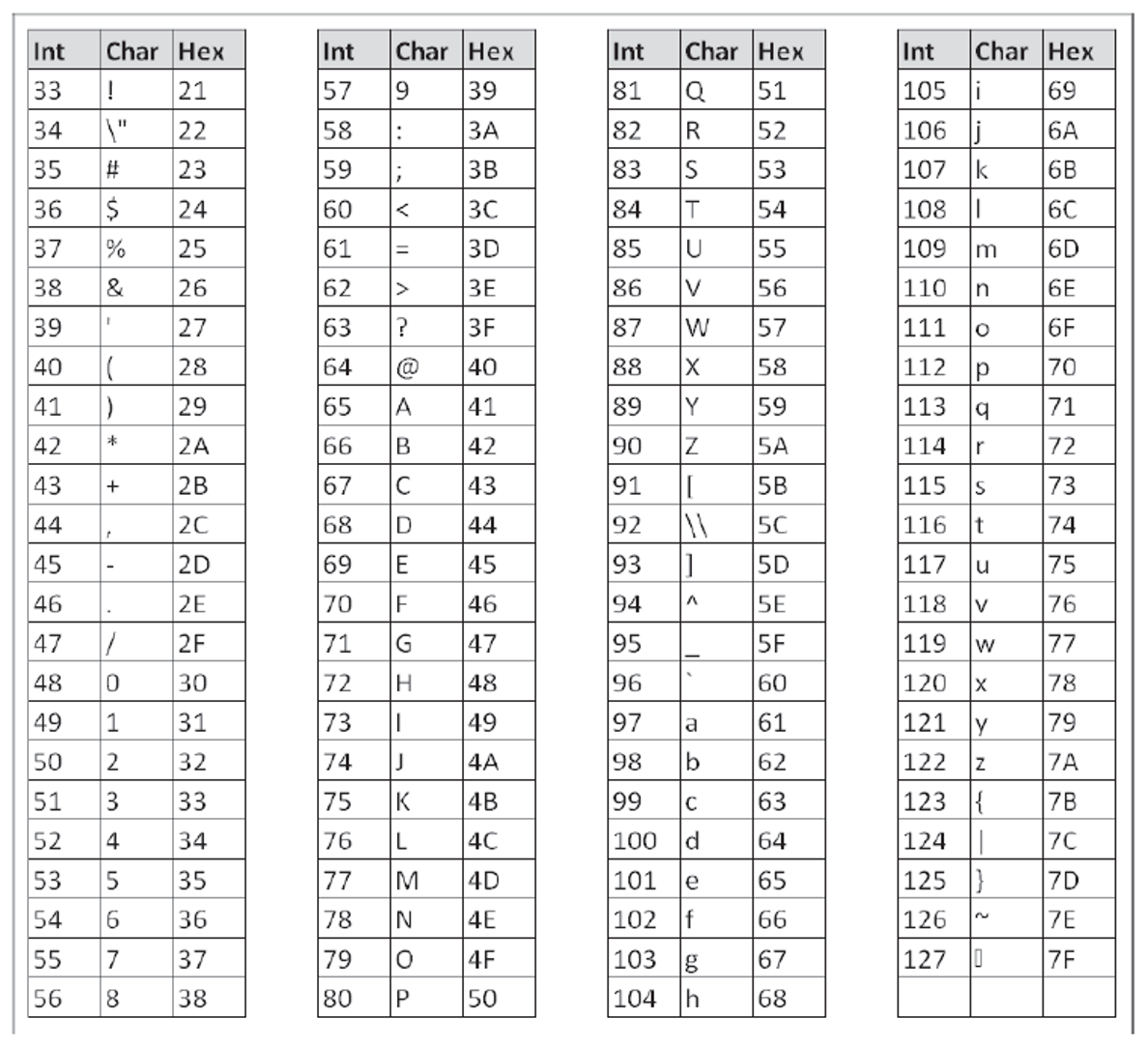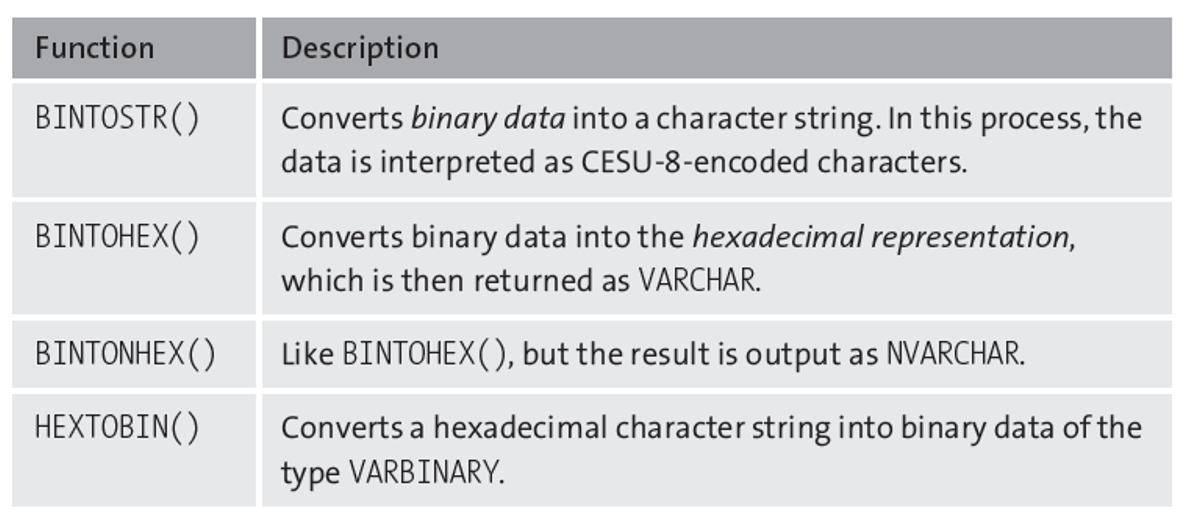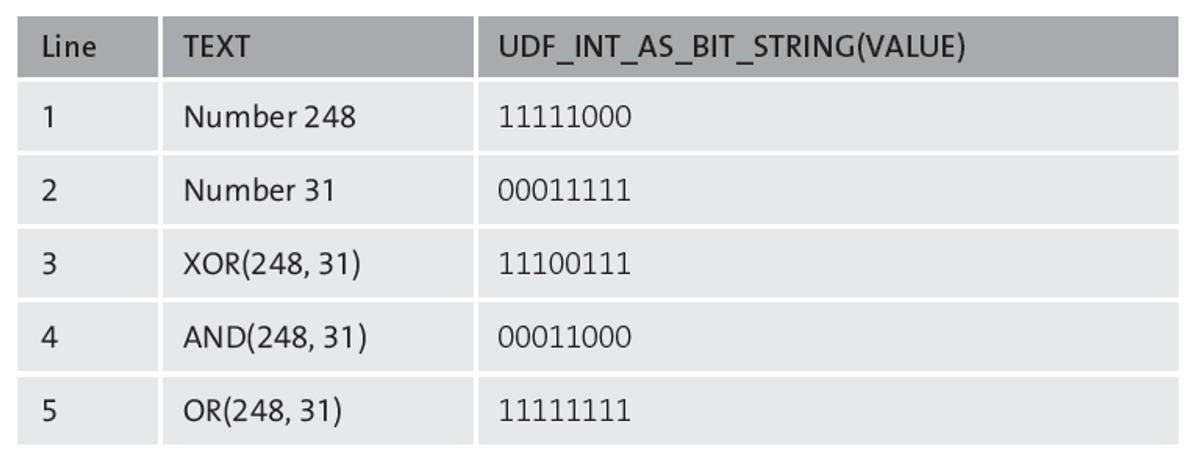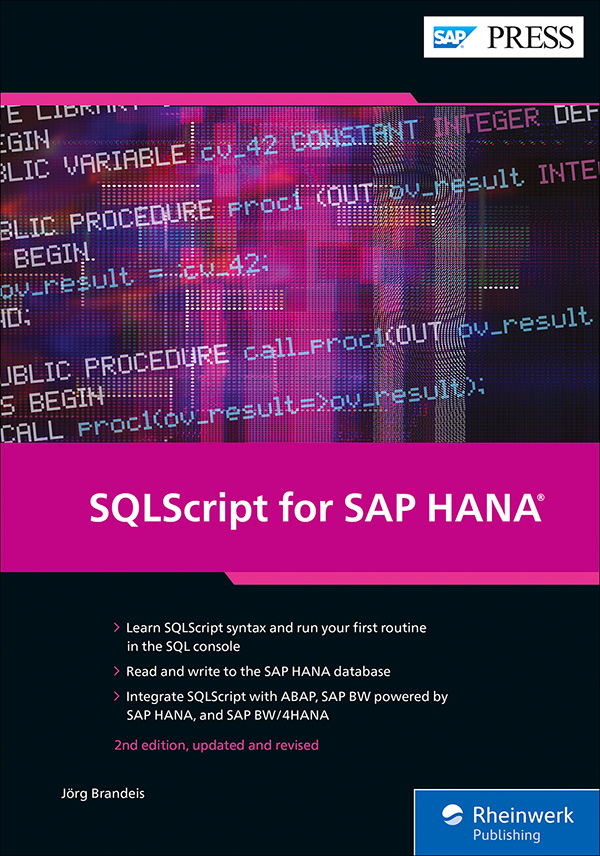In this blog post, we’ll look at different binary data types in SQLScript. If you plan on using the language in your SAP projects, read on!

The data type VARBINARY(n) can store binary data up to a length of 5,000 bytes. The output of a VARBINARY value in the SQL console, or its conversion into a character string, is always in hexadecimal format. If you insert a character string into a column of type VARBINARY, the characters are interpreted as hexadecimal values.

BLOB is a data type for large quantities of binary data. As is the case with other LOB data types, its length is restricted to 2 GB.

To store a binary value as a literal in the program code, the value must be written in hexadecimal notation and with a leading X, as in this simple example:

`SELECT BINTOSTR( X' 48656C6C6F20576F726C64' ) FROM dummy;`

The listing below shows an example of how the VARBINARY data type can be used in the database. To illustrate the properties of the binary data, a value between 33 and 127 is processed per line. The CHAR() function converts these values into the corresponding character from the ASCII character set, which is subsequently converted into a binary value via the TO_VARBINARY() function. The result is an ASCII table, shown after the listing.

`CREATE COLUMN TABLE test_varbin(`

`                            ascii_int INT,`

`                            ascii_char VARCHAR(1),`

`                            ascii_hex VARBINARY(1)) ;`

` `

`DO BEGIN`

` `

`   DECLARE i INT;`

`   FOR i IN 33..127 DO`

`   INSERT INTO test_varbin VALUES(:i,`

`                                  CHAR(:i),`

`                                  BINTONHEX(CHAR(:i))) ;`

`   END FOR;`

`END;`

` `

`SELECT ascii_int AS "Int",`

`               ascii_char AS "Char",`

`               ascii_hex AS "Hex" FROM test_varbin;`

`DROP TABLE test_varbin;`## Conversion between Binary Data, Hexadecimal Data, and Character Strings

The *TO*() functions enable you to convert data between the different formats. The table below shows all conversion functions.## Bits and Bytes

The functions BITSET(<binary data>, <start>, <number>) and BITUNSET(...) enable you to set and delete a number of successive bits from the start in the binary data of type VARBINARY. The first bit is designated as 1. In the following example, the 4 bits are set to the far left in the second byte:

`SELECT bitset(x'0000', 9, 4) from dummy;`

The result is 00 F0.

The functions BITOR(), BITAND(), BITXOR(), and BITNOT() enable you to carry out logical operations bit by bit. The input parameters permitted in these functions are either binary data of type VARBINARY or integers of type INTEGER. The return type corresponds to the data type of the input parameters.

Unfortunately, the individual bits can’t be seen directly either in binary data or in the integers. To improve the readability of the example shown below, we’ll first create a function that represents an 8-bit integer between 0 and 255 in binary notation with eight ones and zeros. The bit with the lowest value is located on the far right. For this conversion, we’ll use imperative functions.

`CREATE FUNCTION udf_int_as_bit_string(iv_value INT)`

`RETURNS rv_value VARCHAR(8)`

`AS BEGIN`

`     DECLARE lv_value INT default :iv_value;`

`     DECLARE lv_mod INT;`

`     rv_value = '';`

` `

`     WHILE lv_value > 0 DO`

`           lv_mod = MOD(:lv_value, 2);`

`           IF lv_mod = 1 THEN`

`             rv_value = '1' || :rv_value;`

`4`

`           ELSE`

`             rv_value = '0' || :rv_value;`

`           END IF;`

`           lv_value = lv_value / 2;`

`     END WHILE;`

`     rv_value = LPAD(rv_value, 8, '0');`

`END;`

The function in below takes advantage of a particular property of integers: In binary representation, the bit with the lowest value determines whether a number is even or odd. So, the input is always divided by 2. If the remainder equals 1, the corresponding bit is set; otherwise, the bit is not set. At the same time, division by 2 means a shift to the right by 1 bit.

`CREATE TABLE binval(text NVARCHAR(50), value INT);`

`INSERT INTO binval VALUES ('Integer 248', 248 );`

`INSERT INTO binval VALUES ('Integer 31', 31 );`

`INSERT INTO binval VALUES ('XOR(248, 31)', BITXOR(248, 31));`

`INSERT INTO binval VALUES ('AND(248, 31)', BITAND(248, 31));`

`INSERT INTO binval VALUES ('OR(248, 31)' , BITOR(248, 31));`

` `

`SELECT text,`

`       udf_int_as_bit_string(value)`

`FROM binval;`

` `

`DROP TABLE binval;`

` `

As shown in above, the numbers 248 and 31 are first inserted into the Bitwise output table, followed by the result of the operations XOR, AND, and OR with both numbers. The bitwise output shown below provides a clear overview of these operations.The BITCOUNT() function allows you to count the number of set bits of an integer or in binary data.

Editor’s note: This post has been adapted from a section of the book SQLScript for SAP HANA by Jörg Brandeis.

## RecommendationSQLScript for SAP HANA

New to SQLScript—or maybe looking to brush up on a specific task? Whatever your skill level, this comprehensive guide to SQLScript for SAP HANA is for you! Master language elements, data types, and the function library. Learn to implement SAP HANA database procedures and functions using imperative and declarative SQLScript. Integrate with ABAP, SAP BW on SAP HANA, and SAP BW/4HANA. Finally, test, troubleshoot, and analyze your SQLScript programs. Code like the pros!